## Day 1, Problem 3: Mhocskian Languages

Linguists are currently studying Mhocskian, the language of the native inhabitants of Mhocsky island. The linguists have found a description of how the natives construct words in Mhocskian, and a list of words. The linguists would now like to know which of the words in the list are valid Mhocskian words.

### Rules

Words in Mhocskian are constructed according to a set of rules. These rules involve two types of components: variables and terminals. A variable is an uppercase letter used in the description of the rules. A terminal is a lowercase letter that is part of a Mhocskian word.

There are two types of rules. The first type of rule allows you to replace a variable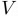$V$ by two variables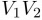$V_{1}V_{2}$ in that order, and we write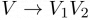$V \rightarrow V_{1}V_{2}$ as a short form for this type of rule. The second type of rule allows you to replace a variable$V$ by a terminal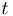$t$, and we write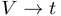$V \rightarrow t$ as a short form for this type of rule.

One of the variables is the start variable. A word w is composed of lowercase letters from the English alphabet. It is a valid Mhocskian word if, starting from the start variable, it is possible to follow a sequence of rules to obtain w.

### Example

Suppose we have variables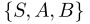$\{S, A, B\}$, terminals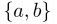$\{a, b\}$, and rules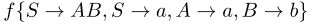$f\{S \rightarrow AB, S \rightarrow a, A \rightarrow a, B \rightarrow b\}$

The word "ab" is a valid Mhocskian word because it can be constructed in the following way: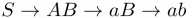$S \rightarrow AB \rightarrow aB \rightarrow ab$. The word "a" can be constructed simply by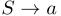$S \rightarrow a$. The word "b" cannot be constructed.

### Input Format

On the first line, two integers V and T in that order.

On the second line, V space separated uppercase letters, the variables. The first variable on the line is always the start variable.

On the third line, T space separated lowercase letters, the terminals.

On the fourth line, there is an integer R1. R1 lines follow, each of which is of the form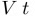$V \: t$, representing a rule$V \rightarrow t$.

On the next line, there is an integer R2. R2 lines follow, each of which is of the form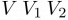$V \: V_1 \: V_2$, representing a rule$V \rightarrow V_{1}V_{2}$.

On the next line, there is an integer W. W lines follow; each contains a single word made entirely of lowercase letters.

### Output Format

The output must contain W lines. On line i, output a 1 if the ith word is a valid Mhocskian word, and 0 otherwise.

### Constraints

1 ≤ V,T ≤ 26

1 ≤ R1 + R2 ≤ 30

1 ≤ W ≤ 20

Each of the words in the linguists' list will have length between 1 and 30.

### Sample Input

5 2
I S A B C
a b
2
A a
B b
7
I A B
I A C
C S B
S A B
S A C
I S S
S S S
4
abababaaabbbaabbaabb
abab
bbaa
aaabababbaaabbbb

### Sample Output

1
1
0
1

Point Value: 20 (partial)
Time Limit: 2.00s
Memory Limit: 64M